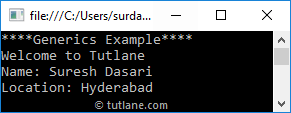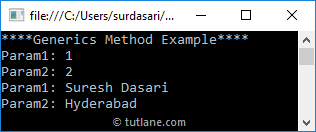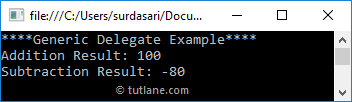# Visual Basic Generics

In visual basic, generic is a type and it is useful to define a class, structure, interface or method with placeholders (type parameters) to indicate that they can store or use one or more of the types. In visual basic, the compiler will replace placeholders with the specified type at compile-time.

In visual basic, mostly we will use generics with collections and the methods that operate on them to specify a type of objects to be stored in a collection. The generics are introduced in .NET Framework 2.0 with a new namespace called System.Collections.Generic

In visual basic, generics are useful to improve the code reusability, type safety, and performance when compared with the non-generic types such as arraylist.

## Visual Basic Generics Declaration

To define a class or method as generic, we need to use the type parameter as a placeholder within the brackets.

Following is the example of defining a generic class with type parameter (T) as a placeholder within the brackets.

Public Class GenericClass(Of T)

Public msg As T

Public Sub genericMethod(ByVal name As T, ByVal location As T)

Console.WriteLine("{0}", msg)

Console.WriteLine("Name: {0}", name)

Console.WriteLine("Location: {0}", location)

End Sub

End Class

If you observe the above class, we created a class (GenericClass) with one parameter (msg) and method (genericMethod) using type parameter (T) as a placeholder with brackets.

Here, (Of type) will indicate GenericClass is generic and type parameter (T) is used to accept a requested type. The type parameter name can be anything like X or U or etc. based on our requirements.

Generally, while creating an instance of the class we need to specify an actual type, then the compiler will replace all the type parameters such as T or U or X, etc. with the specified actual type. In visual basic, the following is the example of creating an instance of a generic class.

' Instantiate Generic Class, string is the type argument

Dim gclass As GenericClass(Of String) = New GenericClass(Of String)()

gclass.msg = "Welcome to Tutlane"

If you observe the above code, we are sending the type as “String”. So, the compiler will substitute all the type parameters (T) with the defined type “String” and our class (GenericClass) will be like as shown below.

Public Class GenericClass

Public msg As String

Public Sub genericMethod(ByVal name As String, ByVal location As String)

Console.WriteLine("{0}", msg)

Console.WriteLine("Name: {0}", name)

Console.WriteLine("Location: {0}", location)

End Sub

End Class

In visual basic, we can also create our own custom generic types and methods to provide our own generalized solutions that are type-safe and efficient.

## Visual Basic Generic Class Example

Following is the example of creating a generic class using type parameter (T) with brackets (Of type) in a visual basic programming language.

Module Module1

Public Class GenericClass(Of T)

Public msg As T

Public Sub genericMethod(ByVal name As T, ByVal location As T)

Console.WriteLine("{0}", msg)

Console.WriteLine("Name: {0}", name)

Console.WriteLine("Location: {0}", location)

End Sub

End Class

Sub Main(ByVal args As String())

Console.WriteLine("****Generics Example****")

' Instantiate Generic Class, string is the type argument

Dim gclass As GenericClass(Of String) = New GenericClass(Of String)()

gclass.msg = "Welcome to Tutlane"

End Sub

End Module

When we execute the above visual basic program, we will get the result like as shown below.This is how we can use the generics in visual basic to create generic classes or methods based on our requirements.

## Visual Basic Generic Class as Base / Derived Class

In visual basic, we can use the generic class as a base class, but we need to provide a type instead of type parameter for the base class because there is no way to send a required type argument to instantiate a base class at run time.

Following is the example of using a generic class as a base class in a visual basic programming language.

' No Error

Class DClass1

Inherits GenericClass(Of String)

' Implementation

End Class

' Compile-time Error

'Class DClass2

' Inherits GenericClass(Of T)

' Implementation

'End Class

In case, if derived class is generic, then we don’t need to specify a type for generic base class instead we can use type parameter (T).

Following is the example of defining a generic derived class in a visual basic programming language.

' No Error

Class DClass1

Inherits GenericClass(Of String)

' Implementation

End Class

' No Error

Class DClass2(Of T)

Inherits GenericClass(Of T)

' Implementation

End Class

## Visual Basic Generic Methods

In visual basic, if we define a method with type parameter, it is called a generic method. Following is the example of defining a generic method with type parameter using brackets (Of type).

Public Sub genericMethod(Of T)(ByVal a As T, ByVal b As T)

' Implementation

End Sub

This generic method can be called either by specifying the type of argument or without an argument like as shown below.

genericMethod(Of Integer)(1, 2)

## Visual Basic Generic Method Example

In visual basic, we can call a generic method bypassing any type of arguments. Following is the example of defining a generic method in visual basic programming language.

Module Module1

Public Class SampleClass

Public Sub GMethod(Of T)(ByVal a As T, ByVal b As T)

Console.WriteLine("Param1: {0}", a)

Console.WriteLine("Param2: {0}", b)

End Sub

End Class

Sub Main(ByVal args As String())

Console.WriteLine("****Generics Method Example****")

Dim s As SampleClass = New SampleClass()

s.GMethod(Of Integer)(1, 2)

End Sub

End Module

If you observe the above code, we are calling our generic method (GMethod) with or without type parameter and sending different types of arguments based on our requirements.

When we execute the above visual basic program, we will get the result like as shown below.This is how we can define generic methods in a visual basic programming language based on our requirements.

## Visual Basic Generic Delegates

In visual basic, the generic delegate is same as a normal delegate but the only difference is the generic delegate will have a generic type with brackets (Of type).

Following is the example of defining a generic delegate in visual basic programming language.

Module Module1

' Declare Generic Delegate

Public Delegate Function SampleDelegate(Of T)(ByVal a As T, ByVal b As T) As T

Class MathOperations

Public Function Add(ByVal a As Integer, ByVal b As Integer) As Integer

Return a + b

End Function

Public Function Subtract(ByVal x As Integer, ByVal y As Integer) As Integer

Return x - y

End Function

End Class

Sub Main(ByVal args As String())

Console.WriteLine("****Generic Delegate Example****")

Dim m As MathOperations = New MathOperations()

' Instantiate delegate with add method

Console.WriteLine("Addition Result: " & dlgt(10, 90))

' Instantiate delegate with subtract method

Console.WriteLine("Subtraction Result: " & dlgt(10, 90))

End Sub

End Module

If you observe the above code, we defined delegate (SampleDelegate) with generic type parameter (T) using brackets (Of type) and accessing it by creating an instance of delegate with the required argument (int).

When we execute the above visual basic program, we will get a result like as shown below.This is how we can use the generics with delegates based on our requirements in a visual basic programming language.

## Visual Basic Generics Overview

Following are the important properties which we need to remember about generics in visual basic programming language.

• In visual basic, generics are represented by using brackets (Of type).
• To define class or method as generic, we need to use type parameter as a placeholder within brackets (Of type).
• The compiler will replace all the placeholders with the specified type at compile time.
• In visual basic, generics are useful to improve the code reusability, type safety and the performance when compared with the non-generic types such as arraylist.
• In visual basic, we can use a generics with classesinterfaces methods, propertiesdelegates, events and with operators.